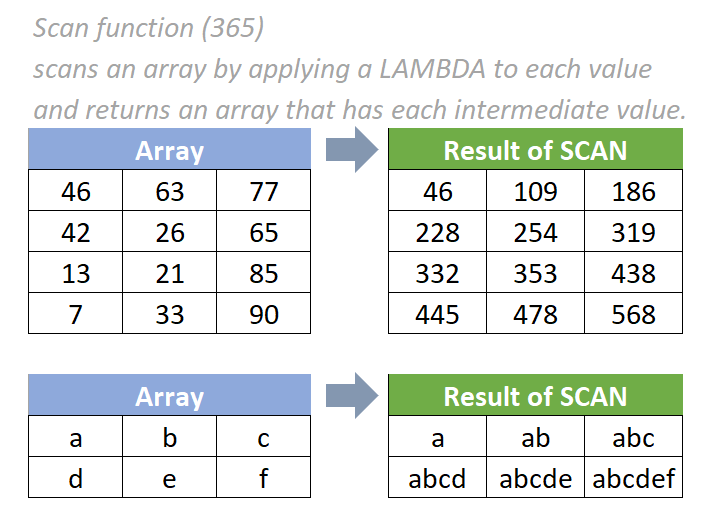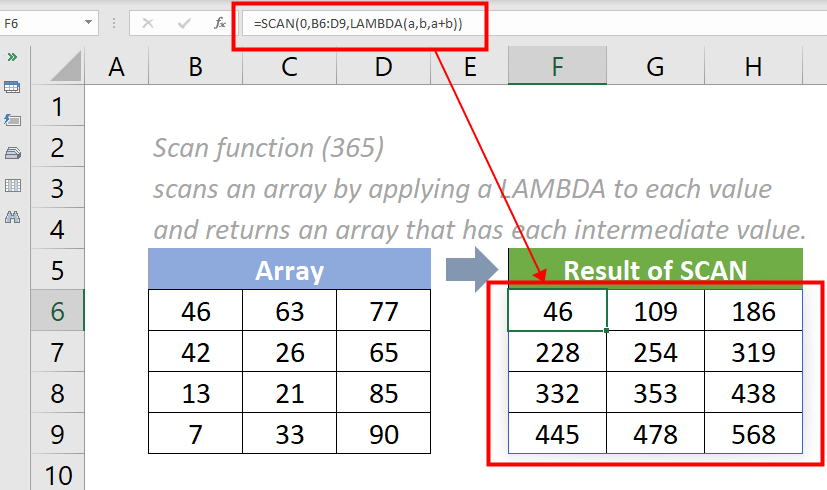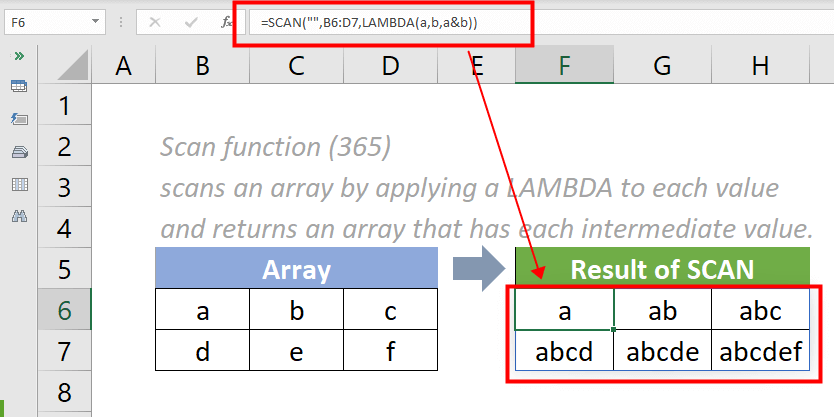## Excel SCAN function (365)

The SCAN function applies a LAMBDA function to each value in an array and returns an array that contains the intermediate values while scanning the array.#### Syntax

=REDUCE ([initial_value],array,lambda(accumulator, value))

#### Arguments

• Initial_value (optional): The starting value of the accumulator.
• Array (required): The array to be scanned.
• Lambda (required): The custom LAMBDA function that is applied to scan the array.
• Accumulator (required): The value totaled up and returned as the final result.
• Value (required): The calculation applied to each element in the array.

#### Return Value

The SCAN function returns an array of results.

#### Function notes

1. The SCAN function is newly introduced in Excel for Microsoft 365. So it is not available in earlier versions of Excel. New dynamic array formulas are introduced in Excel for Microsoft 365, meaning no need to use Ctrl+ Shift+ Enter to enter the SCAN formula as an array formula.
2. The #VALUE! error value occurs if one of the below situations occur:
• an invalid LAMBDA function is provided;
• a wrong number of parameters is provided.
3. The initial_value argument is used to set the starting value for the accumulator parameter. After the first result is returned, the initial_value changes to the result value. And the process iterates over all elements in an array from column to row. If you are working with text, set the initial value to "".

#### Example One:

As shown in the screenshot below, there is an array of data. I want to keep adding each value in a cell and its adjacent cell value, from column to row, please do the following:

Please copy the formula below into cell F6, then press the Enter key to get the result.

=SCAN (0,B6:D9,LAMBDA(a,b,a+b))Note: In the example above, the SCAN Function deals with numbers and the supplied initial_value argument is set to 0. In this case, it can also be omitted and replaced by an empty space. For example, the formula in cell F6 can be changed to:

=SCAN ( ,B6:D9,LAMBDA(a,b,a+b))

#### Example Two:

As shown in the screenshot below, there is an array of data. I want to concatenate characters in the given array, and return an array that contains the intermediate values, please do the following:

Please copy the formula below into cell F6, then press the Enter key to get the result.

=SCAN ("",B6:D7,LAMBDA(a,b,a&b))#### Relative Functions:

• Excel MAP Function
The MAP function applies a LAMBDA function to create a new value and returns an array formed by mapping each value in the supplied array(s) to a new value.

• Excel LAMBDA Function
The Excel LAMBDA function is used to create custom functions that can be reused throughout a workbook.

• Excel Reduce Function
The REDUCE function applies a LAMBDA function to each value in an array and returns the total value in the accumulator, reducing the array to an accumulated value.